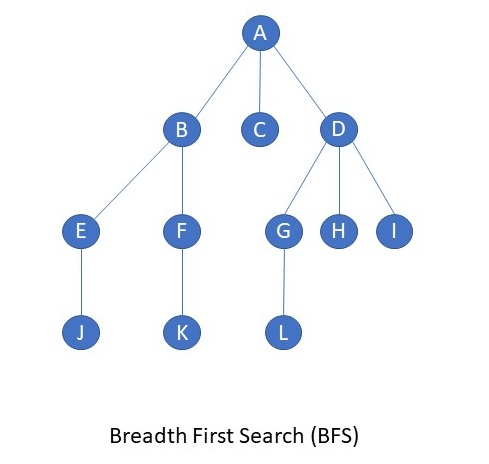# C program to implement Depth First Search(DFS)

C program to implement Depth First Search(DFS). Depth First Search is an algorithm used to search the Tree or Graph. DFS search starts from root node then traversal into left child node and continues, if item found it stops other wise it continues. The advantage of DFS is it requires less memory compare to Breadth First Search(BFS).Read more about C Programming Language .

`/************************************************************ You can use all the programs on  www.c-program-example.com* for personal and learning purposes. For permissions to use the* programs for commercial purposes,* contact [email protected]* To find more C programs, do visit www.c-program-example.com* and browse!* *                      Happy Coding***********************************************************/#include<stdio.h>#include<conio.h>int a,reach,n;void dfs(int v){ int i; reach[v]=1; for(i=1;i<=n;i++)  if(a[v][i] && !reach[i])  {   printf("n %d->%d",v,i);   dfs(i);  }}void main(){ int i,j,count=0; clrscr(); printf("n Enter number of vertices:"); scanf("%d",&n); for(i=1;i<=n;i++) {  reach[i]=0;  for(j=1;j<=n;j++)   a[i][j]=0; } printf("n Enter the adjacency matrix:n"); for(i=1;i<=n;i++)  for(j=1;j<=n;j++)   scanf("%d",&a[i][j]); dfs(1); printf("n"); for(i=1;i<=n;i++) {  if(reach[i])   count++; } if(count==n)  printf("n Graph is connected"); else  printf("n Graph is not connected"); getch();`
`Read more Similar C ProgramsData StructuresBreadth First Search(BFS)Learn C Programming`

You can easily select the code by double clicking on the code area above.

You can discuss these programs on our Facebook Page. Start a discussion right now,

our page!

(you can send this program to your friend using this button)

Like to get updates right inside your feed reader? Grab our feed!

(c) www.c-program-example.com

# Breadth First Search (BFS) in C

### Breadth First Search/Traversal

C program to implement Breadth First Search(BFS). Breadth First Search is an algorithm used to search a Tree or Graph. BFS search starts from root node then traverses into next level of graph or tree, if item found it stops other wise it continues with other nodes in the same level before moving on to the next level. The algorithm can also be used for just Tree/Graph traversal, without actually searching for a value. This is what being done in the program below. The disadvantage of BFS is it requires more memory compare to Depth First Search(DFS).### The Program

 #include int a, q, visited, n, i, j, f = 0, r = -1; void bfs(int v) { for(i = 1; i <= n; i++) if(a[v][i] && !visited[i]) q[++r] = i; if(f <= r) { visited[q[f]] = 1; bfs(q[f++]); } } void main() { int v; printf("\n Enter the number of vertices:"); scanf("%d", &n); for(i=1; i <= n; i++) { q[i] = 0; visited[i] = 0; } printf("\n Enter graph data in matrix form:\n"); for(i=1; i<=n; i++) { for(j=1;j<=n;j++) { scanf("%d", &a[i][j]); } } printf("\n Enter the starting vertex:"); scanf("%d", &v); bfs(v); printf("\n The node which are reachable are:\n"); for(i=1; i <= n; i++) { if(visited[i]) printf("%d\t", i); else { printf("\n Bfs is not possible. Not all nodes are reachable"); break; } } }
view raw bfs.c hosted with ❤ by GitHub